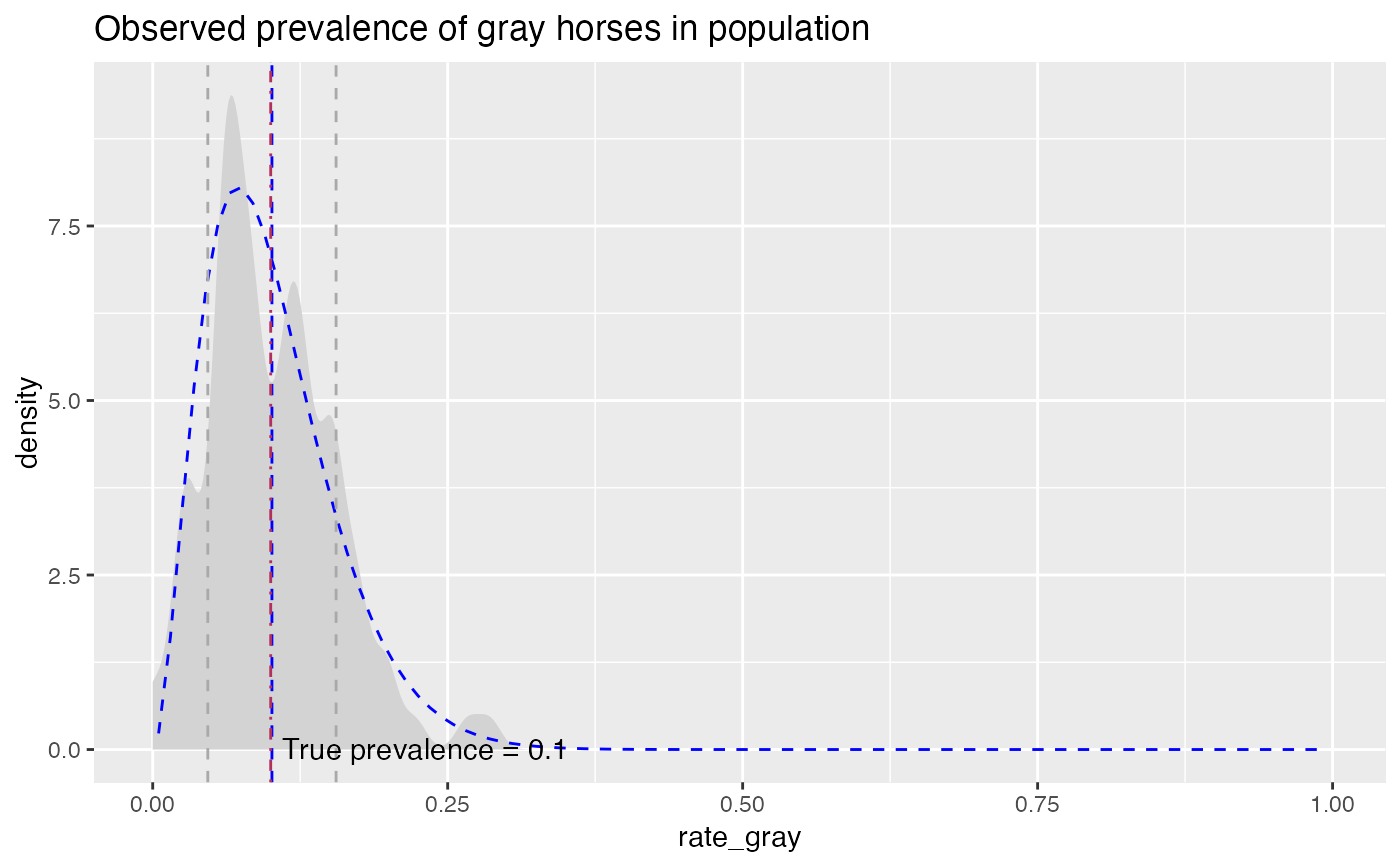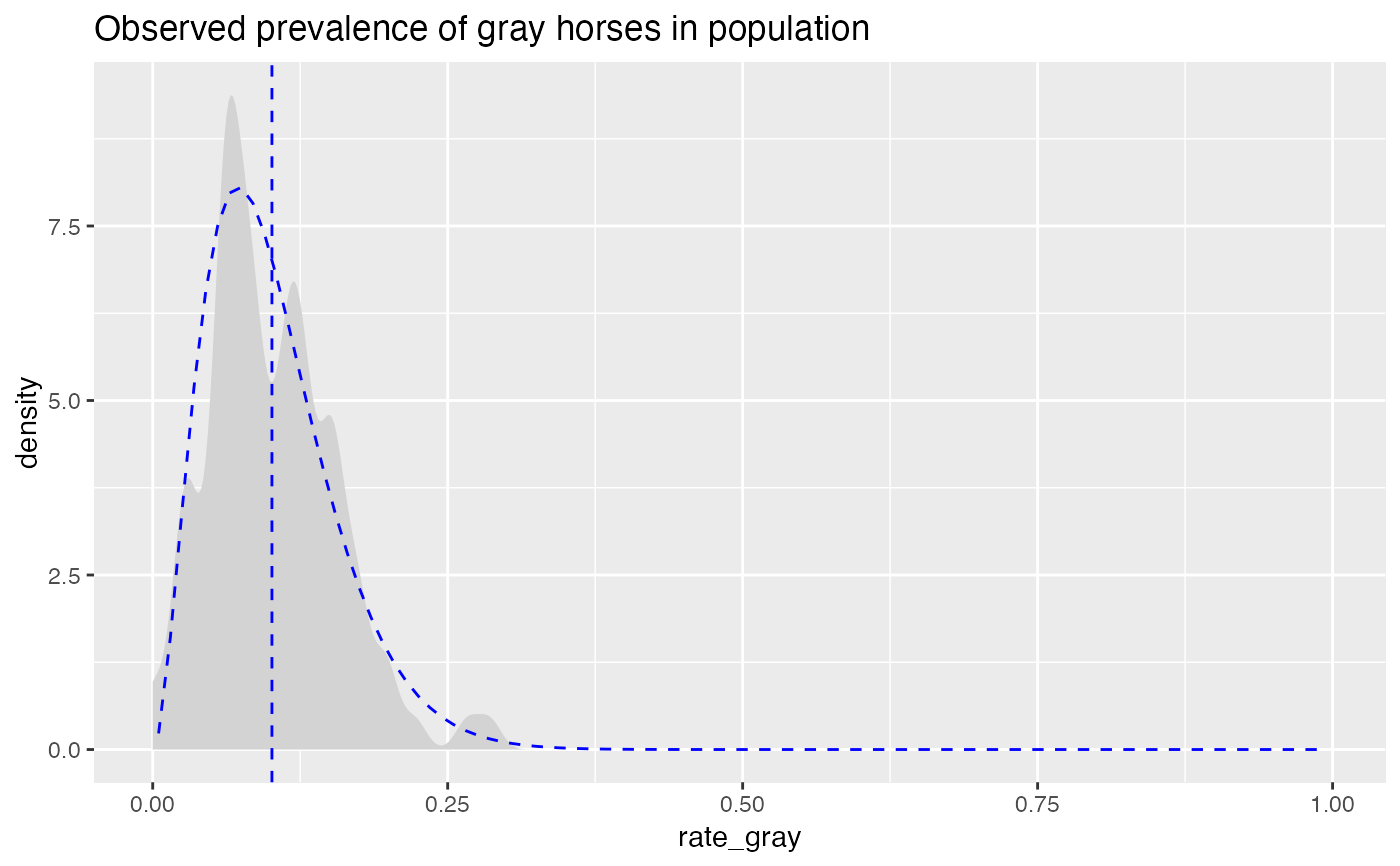Compares empirical rate data to a beta distribution with the same mean and standard deviation.

PlotDistDensityBeta(
frm,
xvar,
title,
...,
curve_color = "lightgray",
beta_color = "blue",
mean_color = "blue",
sd_color = "darkgray"
)

## Arguments

frm data frame to get values from name of the independent (input or model) column in frame title to place on plot force later arguments to bind by name color for empirical density curve color for matching theoretical beta color for mean line color for 1-standard deviation lines (can be NULL)

## Details

Plots the empirical density, the theoretical matching beta, the mean value, and plus/minus one standard deviation from the mean.

## Examples


set.seed(52523)
N = 100
pgray = 0.1  # rate of gray horses in the population
herd_size = round(runif(N, min=25, 50))
ngray = rbinom(N, herd_size, pgray)
hdata = data.frame(n_gray=ngray, herd_size=herd_size)

# observed rate of gray horses in each herd
hdata\$rate_gray = with(hdata, ngray/herd_size)

title = "Observed prevalence of gray horses in population"

PlotDistDensityBeta(hdata, "rate_gray", title) +
ggplot2::geom_vline(xintercept = pgray, linetype=4, color="maroon") +
ggplot2::annotate("text", x=pgray+0.01, y=0.01, hjust="left",
label = paste("True prevalence =", pgray))# no sd lines
PlotDistDensityBeta(hdata, "rate_gray", title,
sd_color=NULL)